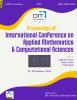# The Pollutant Concentration Regime in a Flow due to Variable Time-Dependent Off-Diagonal Dispersion

## Authors

O R Jimoh
Department of Mathematics, Federal University of Technology, Minna, Nigeria
Y M Aiyesimi
Department of Mathematics, Federal University of Technology, Minna, Nigeria

### Synopsis

In this paper, an Eigen Functions expansion technique was used to obtain an analytical solution of two dimensional contaminant flow problem with non-zero initial concentration. The equation which describes the two-dimensional contaminant flow model is a partial differential equation characterized by advection, dispersion, adsorption, first order decay and zero-order source. It was assumed that the adsorption term was modeled by Freudlich isotherm. The off-diagonal dispersion parameter was incorporated into the two-dimensional contaminant model in order to expand the scope of the analysis. The model equation was non-dimensionalized before the parameter expanding method was applied. The resulting equations were solved successively by Eigen functions expansion technique. This research establishes that the pollutant concentration declines with increase in distances in both directions as the off-diagonal dispersion coefficient, zero-order source coefficient and vertical dispersion coefficient increases.Published
August 8, 2020
Series
Online ISSN
2582-3922